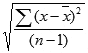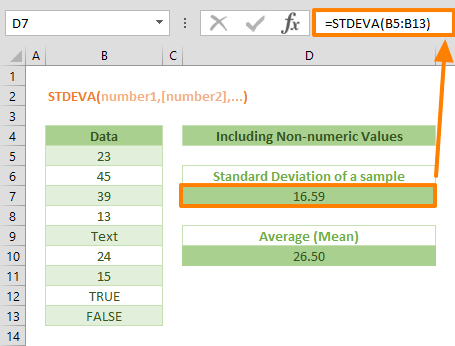The STDEVA is a Statistical function that calculates and returns the standard deviation for a sample data. The standard deviation is a measure of how widely values are dispersed from the average value (the mean). The STDEVA function calculates standard deviation using the "n-1" method by including text and logical values in the calculation as well. In this guide, we’re going to show you how to use the STDEVA function and also go over some tips and error handling methods.

## Supported versions

• All Excel versions

## DTDEVA Syntax

STDEVA(number1,[number2],...)

## Arguments

 number1 The first number or reference in the sample of the population. You can also use an array instead of multiple arguments. The function evaluates text and logical values with following rules TRUE = 1 FALSE = 0 Any string = 0 [number2], … Other numbers of the population up to 254 arguments (Excel 2007 or later). Other numbers of the population up to 29 arguments (Excel 2003).

## Example

The standard deviation is a measure expressed by how much the members of a group differ from the mean (average) value of that group. The STDEVA function assumes that the given arguments are a sample of a population. The function uses the following formula:where x is the average (mean) and n is the sample size.

All you need to do is to enter your data set for the arguments.

=STDEVA(B5:B13)## Tips

• Use the STDEVA to calculate the standard deviation for a sample of the population by take non-numeric values into calculation.
• The standard deviation is calculated using the "n-1" method.
• Prefer using the STDEV.P to calculate the standard deviation for a sample of the population. The STDEV.P function uses "n" method.
• You can use the AVERAGE function to calculate the mean of a data set.
• Arguments can be numbers or names, arrays, or references that contain numbers.
• Logical values, and text representations of numbers that you type directly into the list of arguments are included in the calculations.
• If an argument is an array or reference, only numbers in that array or reference are counted. Empty cells, logical values, text, or error values in the array or reference are ignored.
• Arguments that are error values or text that cannot be translated into numbers cause errors.
• If you want to exclude logical values and text representations of numbers in a reference as part of the calculation, use the STDEV function.